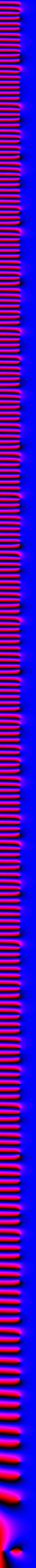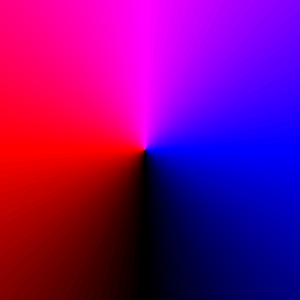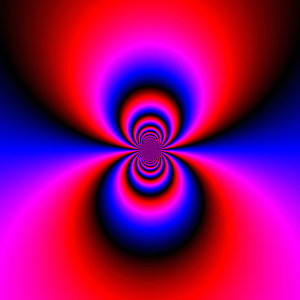## Page 2: The Maclaurin series of exp(z)

 The function f(z) = exp(z) is special because it has no zeros or poles on the complex plane, yet it is not a constant function. How is this possible since the partial sums of its Maclaurin series are polynomials, hence have zeros? The only possibility is that when a term of the series is added it adds one more zero, but it also slightly push every zero away. At the limit, no zero is left because they all have been pushed to infinity. This page shows the process.f(z) = 1. (1 term of the series)f(z) = 1 + z. (2 terms)f(z) = 1 + z + z^2/2. (3 terms)f(z) = 1 + z + z^2/2 + z^3/6. (4 terms)(10 terms)(20 terms)(40 terms)(80 terms)f(z) = exp(z). (limit)f(z) = exp(100/z). We can see what is happening at infinity by "inverting" the complex plane. What you see is called an essential singularity.

page last updated: March 16, 2002